Question

A street light is mounted at the top of a 15-ft-tall pole. A man 6 ft tall walks away from the pole with a speed of 4 ft/s along a straight path. How fast is the tip of his shadow moving when he is 35 ft from the pole?

1.bonexptip

Explanation:

height of pole = 15 ft

height of man = 6 ft

Let the length of shadow is y .

According to the diagram

Let at any time the distance of man is x.

The two triangles are similar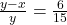15 y – 15 x = 6 y

9 y = 15 x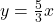Differentiate with respect to time.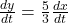As given, dx/dt = 4 ft/s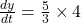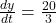ft/s